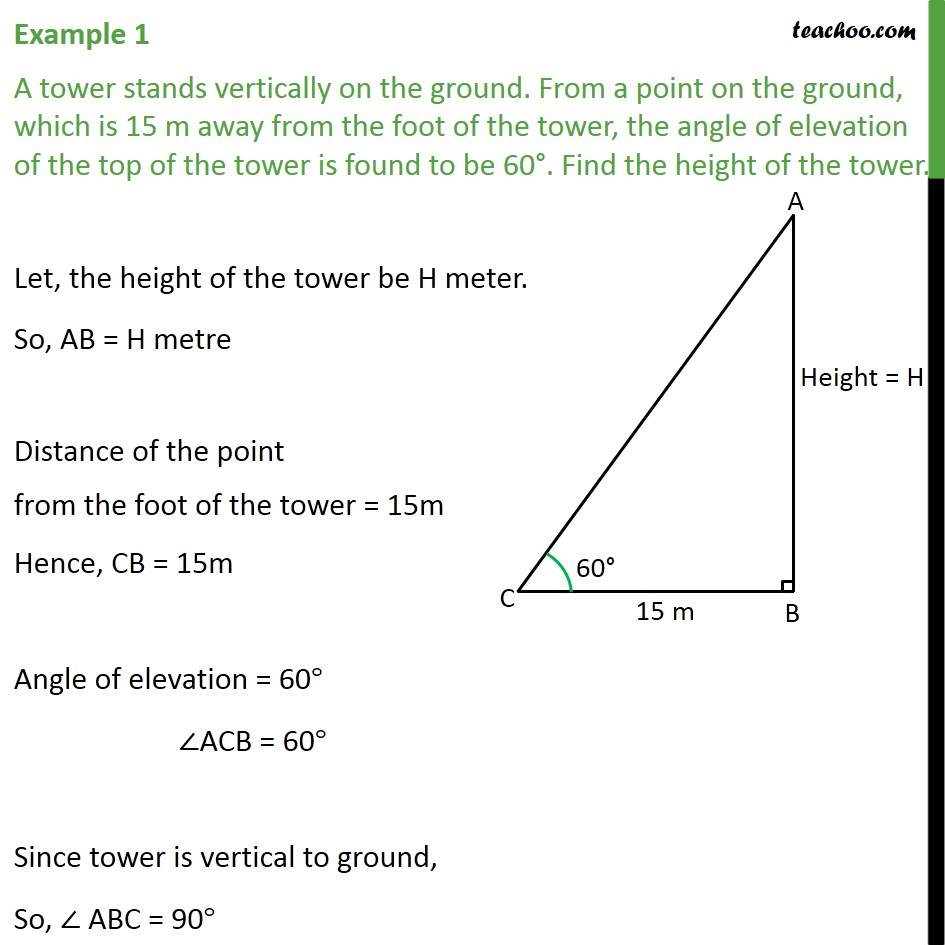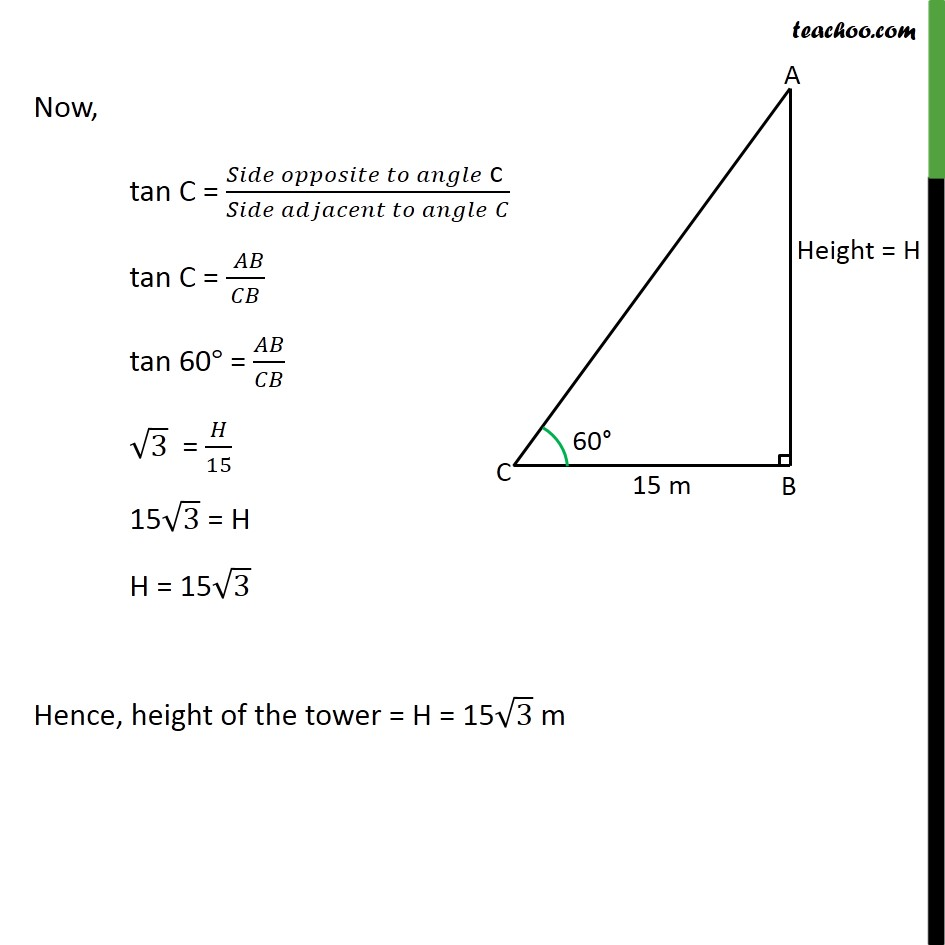1. Chapter 9 Class 10 Some Applications of Trigonometry (Term 2)
2. Concept wise
3. Questions easy to difficult

Transcript

Example 1 A tower stands vertically on the ground. From a point on the ground, which is 15 m away from the foot of the tower, the angle of elevation of the top of the tower is found to be 60°. Find the height of the tower. Let, the height of the tower be H meter. So, AB = H metre Distance of the point from the foot of the tower = 15m Hence, CB = 15m Angle of elevation = 60° ∠ACB = 60° Since tower is vertical to ground, So, ∠ ABC = 90° Now, tan C = (𝑆𝑖𝑑𝑒 𝑜𝑝𝑝𝑜𝑠𝑖𝑡𝑒 𝑡𝑜 𝑎𝑛𝑔𝑙𝑒" c " )/(𝑆𝑖𝑑𝑒 𝑎𝑑𝑗𝑎𝑐𝑒𝑛𝑡 𝑡𝑜 𝑎𝑛𝑔𝑙𝑒" " 𝐶) tan C = (" " 𝐴𝐵)/𝐶𝐵 tan 60° = 𝐴𝐵/𝐶𝐵 √3 = 𝐻/15 15√3 = H H = 15√3 Hence, height of the tower = H = 15√3 m